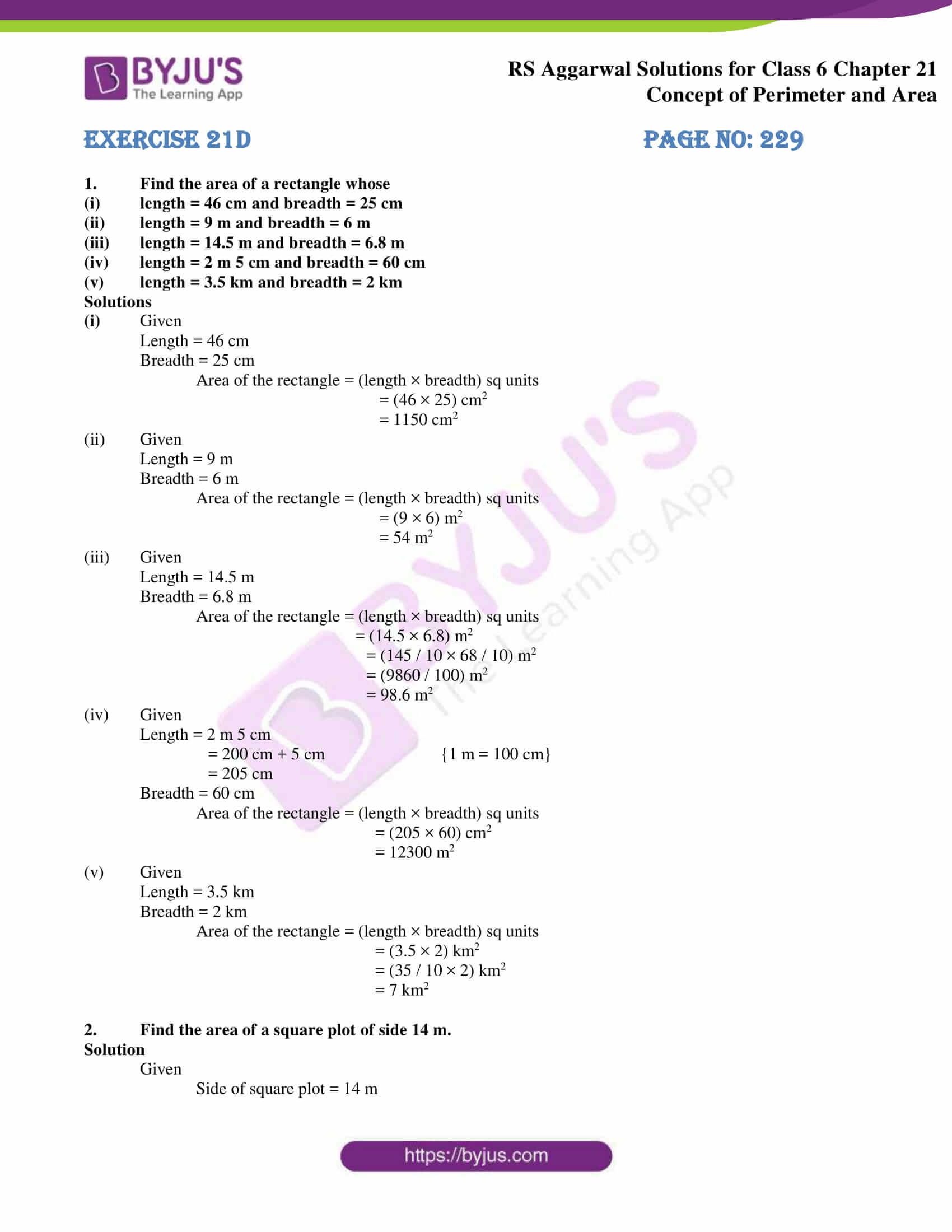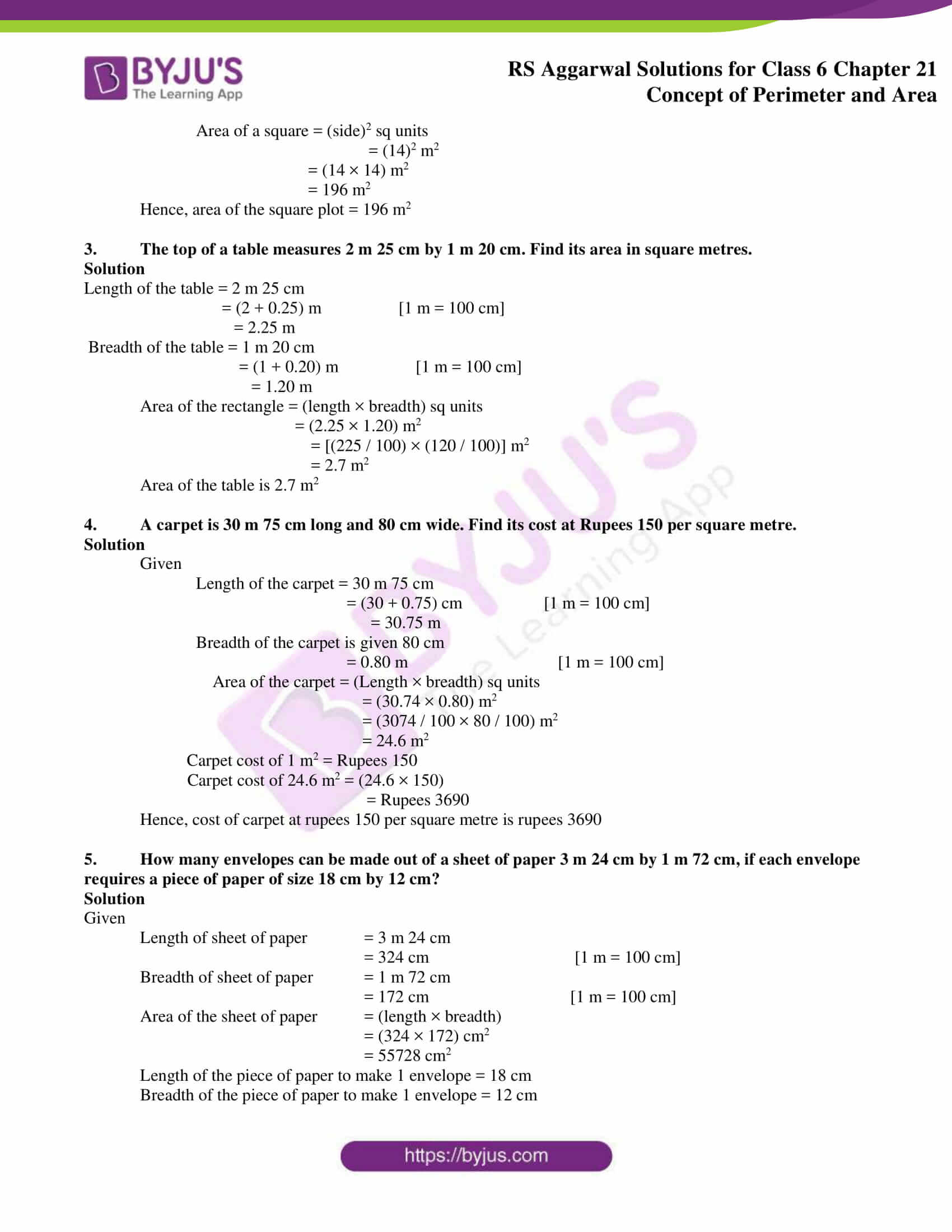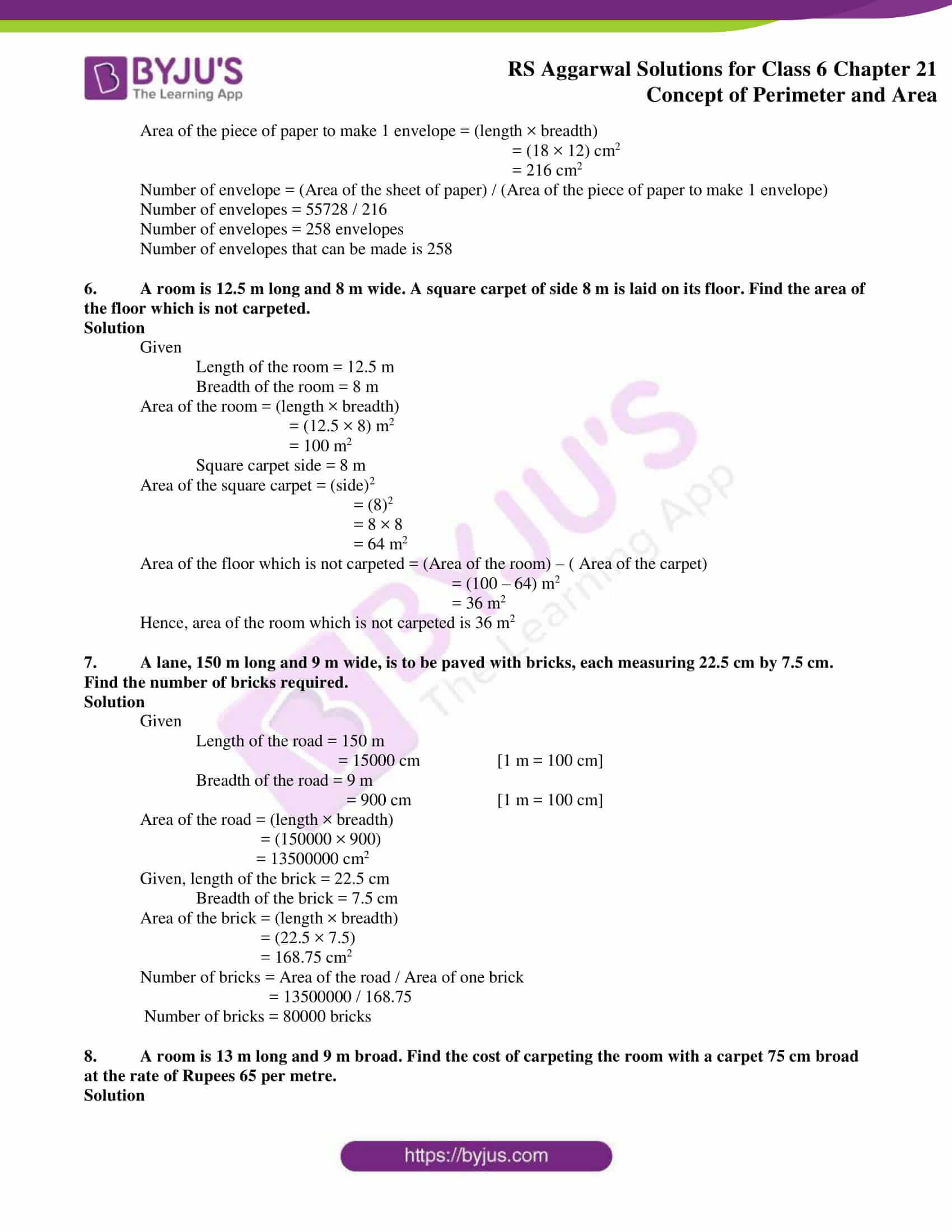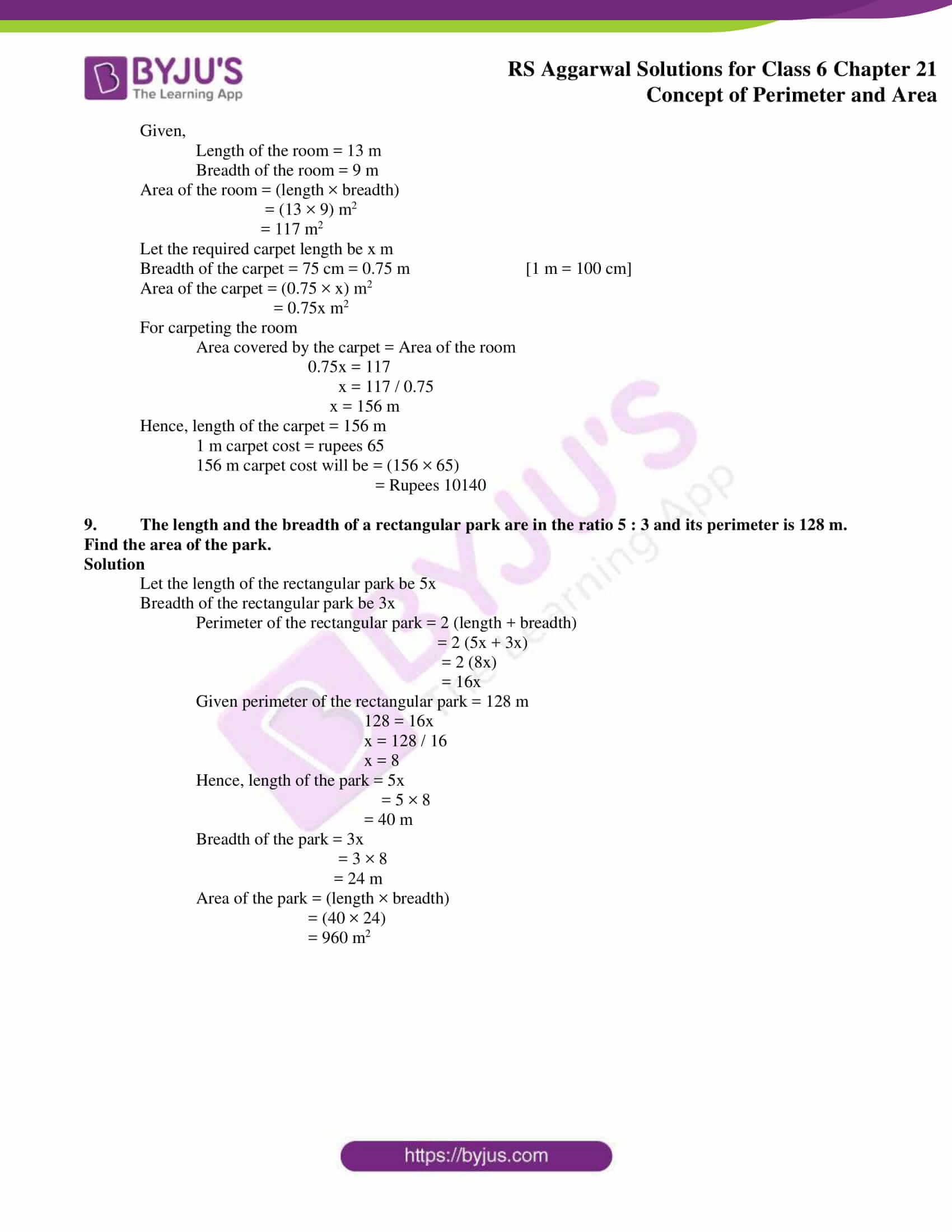# RS Aggarwal Solutions for Class 6 Chapter 21 Concept of Perimeter and Area Exercise 21D

The students can improve their speed in solving exercise wise problems using RS Aggarwal Solutions prepared by experts. The solutions contain explanations in simple language which help in faster grasping of the concept among students. The exercise-wise problems can be solved more quickly with the help of solutions which is significant for the exam. RS Aggarwal Solutions for Class 6 Chapter 21 Concept of Perimeter and Area Exercise 21D PDF can be accessed here for further reference.

## Download PDF of RS Aggarwal Solutions for Class 6 Chapter 21 Concept of Perimeter and Area Exercise 21D### Access answers to Maths RS Aggarwal Solutions for Class 6 Chapter 21 Concept of Perimeter and Area Exercise 21D

1. Find the area of a rectangle whose

(i) length = 46 cm and breadth = 25 cm

(ii) length = 9 m and breadth = 6 m

(iii) length = 14.5 m and breadth = 6.8 m

(iv) length = 2 m 5 cm and breadth = 60 cm

(v) length = 3.5 km and breadth = 2 km

Solutions

(i) Given

Length = 46 cm

Area of the rectangle = (length × breadth) sq units

= (46 × 25) cm2

= 1150 cm2

(ii) Given

Length = 9 m

Area of the rectangle = (length × breadth) sq units

= (9 × 6) m2

= 54 m2

(iii) Given

Length = 14.5 m

Area of the rectangle = (length × breadth) sq units

= (14.5 × 6.8) m2

= (145 / 10 × 68 / 10) m2

= (9860 / 100) m2

= 98.6 m2

(iv) Given

Length = 2 m 5 cm

= 200 cm + 5 cm {1 m = 100 cm}

= 205 cm

Area of the rectangle = (length × breadth) sq units

= (205 × 60) cm2

= 12300 m2

(v) Given

Length = 3.5 km

Area of the rectangle = (length × breadth) sq units

= (3.5 × 2) km2

= (35 / 10 × 2) km2

= 7 km2

2. Find the area of a square plot of side 14 m.

Solution

Given

Side of square plot = 14 m

Area of a square = (side)2 sq units

= (14)2 m2

= (14 × 14) m2

= 196 m2

Hence, area of the square plot = 196 m2

3. The top of a table measures 2 m 25 cm by 1 m 20 cm. Find its area in square metres.

Solution

Length of the table = 2 m 25 cm

= (2 + 0.25) m [1 m = 100 cm]

= 2.25 m

Breadth of the table = 1 m 20 cm

= (1 + 0.20) m [1 m = 100 cm]

= 1.20 m

Area of the rectangle = (length × breadth) sq units

= (2.25 × 1.20) m2

= [(225 / 100) × (120 / 100)] m2

= 2.7 m2

Area of the table is 2.7 m2

4. A carpet is 30 m 75 cm long and 80 cm wide. Find its cost at Rupees 150 per square metre.

Solution

Given

Length of the carpet = 30 m 75 cm

= (30 + 0.75) cm [1 m = 100 cm]

= 30.75 m

Breadth of the carpet is given 80 cm

= 0.80 m [1 m = 100 cm]

Area of the carpet = (Length × breadth) sq units

= (30.74 × 0.80) m2

= (3074 / 100 × 80 / 100) m2

= 24.6 m2

Carpet cost of 1 m2 = Rupees 150

Carpet cost of 24.6 m2 = (24.6 × 150)

= Rupees 3690

Hence, cost of carpet at rupees 150 per square metre is rupees 3690

5. How many envelopes can be made out of a sheet of paper 3 m 24 cm by 1 m 72 cm, if each envelope requires a piece of paper of size 18 cm by 12 cm?

Solution

Given

Length of sheet of paper = 3 m 24 cm

= 324 cm [1 m = 100 cm]

Breadth of sheet of paper = 1 m 72 cm

= 172 cm [1 m = 100 cm]

Area of the sheet of paper = (length × breadth)

= (324 × 172) cm2

= 55728 cm2

Length of the piece of paper to make 1 envelope = 18 cm

Breadth of the piece of paper to make 1 envelope = 12 cm

Area of the piece of paper to make 1 envelope = (length × breadth)

= (18 × 12) cm2

= 216 cm2

Number of envelope = (Area of the sheet of paper) / (Area of the piece of paper to make 1 envelope)

Number of envelopes = 55728 / 216

Number of envelopes = 258 envelopes

Number of envelopes that can be made is 258

6. A room is 12.5 m long and 8 m wide. A square carpet of side 8 m is laid on its floor. Find the area of the floor which is not carpeted.

Solution

Given

Length of the room = 12.5 m

Breadth of the room = 8 m

Area of the room = (length × breadth)

= (12.5 × 8) m2

= 100 m2

Square carpet side = 8 m

Area of the square carpet = (side)2

= (8)2

= 8 × 8

= 64 m2

Area of the floor which is not carpeted = (Area of the room) – ( Area of the carpet)

= (100 – 64) m2

= 36 m2

Hence, area of the room which is not carpeted is 36 m2

7. A lane, 150 m long and 9 m wide, is to be paved with bricks, each measuring 22.5 cm by 7.5 cm. Find the number of bricks required.

Solution

Given

Length of the road = 150 m

= 15000 cm [1 m = 100 cm]

= 900 cm [1 m = 100 cm]

= (150000 × 900)

= 13500000 cm2

Given, length of the brick = 22.5 cm

Breadth of the brick = 7.5 cm

Area of the brick = (length × breadth)

= (22.5 × 7.5)

= 168.75 cm2

Number of bricks = Area of the road / Area of one brick

= 13500000 / 168.75

Number of bricks = 80000 bricks

8. A room is 13 m long and 9 m broad. Find the cost of carpeting the room with a carpet 75 cm broad at the rate of Rupees 65 per metre.

Solution

Given,

Length of the room = 13 m

Breadth of the room = 9 m

Area of the room = (length × breadth)

= (13 × 9) m2

= 117 m2

Let the required carpet length be x m

Breadth of the carpet = 75 cm = 0.75 m [1 m = 100 cm]

Area of the carpet = (0.75 × x) m2

= 0.75x m2

For carpeting the room

Area covered by the carpet = Area of the room

0.75x = 117

x = 117 / 0.75

x = 156 m

Hence, length of the carpet = 156 m

1 m carpet cost = rupees 65

156 m carpet cost will be = (156 × 65)

= Rupees 10140

9. The length and the breadth of a rectangular park are in the ratio 5 : 3 and its perimeter is 128 m. Find the area of the park.

Solution

Let the length of the rectangular park be 5x

Breadth of the rectangular park be 3x

Perimeter of the rectangular park = 2 (length + breadth)

= 2 (5x + 3x)

= 2 (8x)

= 16x

Given perimeter of the rectangular park = 128 m

128 = 16x

x = 128 / 16

x = 8

Hence, length of the park = 5x

= 5 × 8

= 40 m

Breadth of the park = 3x

= 3 × 8

= 24 m

Area of the park = (length × breadth)

= (40 × 24)

= 960 m2

### Access other exercises of RS Aggarwal Solutions for Class 6 Chapter 21 Concept of Perimeter and Area

Exercise 21E Solutions